Documentation

## Rational Polynomials

Rational models are defined as ratios of polynomials and are given by

`$y=\frac{\sum _{i=1}^{n+1}{p}_{i}{x}^{n+1-i}}{{x}^{m}+\sum _{i=1}^{m}{q}_{i}{x}^{m-1}}$`

where n is the degree of the numerator polynomial and 0 ≤ n ≤ 5, while m is the degree of the denominator polynomial and 1 ≤ m ≤ 5. Note that the coefficient associated with xm is always 1. This makes the numerator and denominator unique when the polynomial degrees are the same.

In this guide, rationals are described in terms of the degree of the numerator/the degree of the denominator. For example, a quadratic/cubic rational equation is given by

`$y=\frac{{p}_{1}{x}^{2}+{p}_{2}x+{p}_{3}}{{x}^{3}+{q}_{1}{x}^{2}+{q}_{2}x+{q}_{3}}$`

Like polynomials, rationals are often used when a simple empirical model is required. The main advantage of rationals is their flexibility with data that has a complicated structure. The main disadvantage is that they become unstable when the denominator is around 0. For an example that uses rational polynomials of various degrees, see Example: Rational Fit.

### Fit Rational Models Interactively

1. Open the Curve Fitting app by entering `cftool`. Alternatively, click Curve Fitting on the Apps tab.

2. In the Curve Fitting app, select curve data (X data and Y data, or just Y data against index).

Curve Fitting app creates the default curve fit, `Polynomial`.

3. Change the model type from `Polynomial` to `Rational`.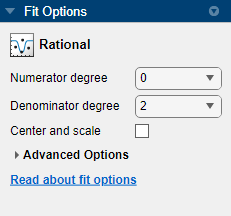You can specify the following options:

• Choose the degree of the numerator and denominator polynomials. The numerator can have degree `0` to `5`, and the denominator from `1` to `5`.

Look in the Results pane to see the model terms, the values of the coefficients, and the goodness-of-fit statistics.

• (Optional) Click Fit Options to specify coefficient starting values and constraint bounds, or change algorithm settings.

The toolbox calculates random start points for rational models, defined on the interval [0,1]. You can override the start points and specify your own values in the Fit Options dialog box.

For more information on the settings, see Specifying Fit Options and Optimized Starting Points.

### Selecting a Rational Fit at the Command Line

Specify the model type `ratij`, where i is the degree of the numerator polynomial and j is the degree of the denominator polynomial. For example, `'rat02'`, `'rat21'` or `'rat55'`.

For example, to load some data and fit a rational model:

```load hahn1; f = fit( temp, thermex, 'rat32') plot(f,temp,thermex)```

See Example: Rational Fit to fit this example interactively with various rational models.

If you want to modify fit options such as coefficient starting values and constraint bounds appropriate for your data, or change algorithm settings, see the table of additional properties with `NonlinearLeastSquares` on the `fitoptions` reference page.

### Example: Rational Fit

This example fits measured data using a rational model. The data describes the coefficient of thermal expansion for copper as a function of temperature in degrees kelvin.

For this data set, you will find the rational equation that produces the best fit. Rational models are defined as a ratio of polynomials as given by:

`$y=\frac{{p}_{1}{x}^{n}+{p}_{2}{x}^{n-1}+...+{p}_{n+1}}{{x}^{m}+{q}_{1}{x}^{m-1}+...+{q}_{m}}$`

where n is the degree of the numerator polynomial and m is the degree of the denominator polynomial. Note that the rational equations are not associated with physical parameters of the data. Instead, they provide a simple and flexible empirical model that you can use for interpolation and extrapolation.

1. Load the thermal expansion data from the file `hahn1.mat`, which is provided with the toolbox.

`load hahn1`

The workspace contains two new variables:

• `temp` is a vector of temperatures in degrees kelvin.

• `thermex` is a vector of thermal expansion coefficients for copper.

2. Open the Curve Fitting app by entering:

`cftool`

3. Select `temp` and `thermex` from the X data and Y data lists.

The Curve Fitting app fits and plots the data.

4. Select `Rational` in the fit category list.

5. Try an initial choice for the rational model of quadratic/quadratic. Select `2` for both Numerator degree and Denominator degree.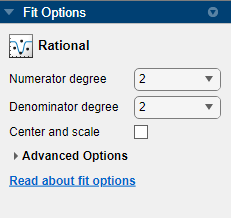6. Examine the residuals. Select View > Residuals Plot or click the toolbar button.

Examine the data, fit, and residuals. Observe that the fit misses the data for the smallest and largest predictor values. Additionally, the residuals show a strong pattern throughout the entire data set, indicating that a better fit is possible.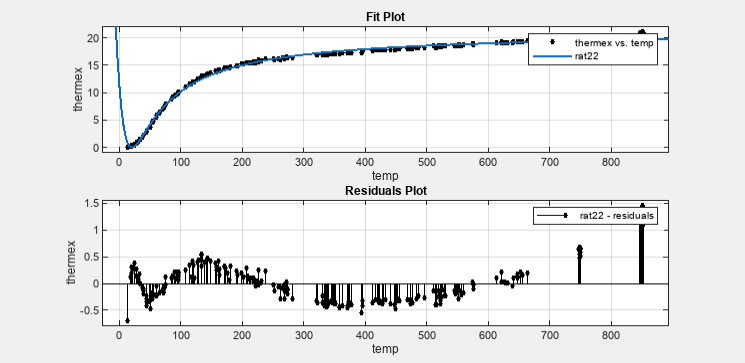7. For the next fit, try a cubic/cubic equation. Select `3` for both Numerator degree and Denominator degree.

Examine the data, fit, and residuals. The fit exhibits several discontinuities around the zeros of the denominator.

### Note

Your results depend on random start points and may vary from those shown.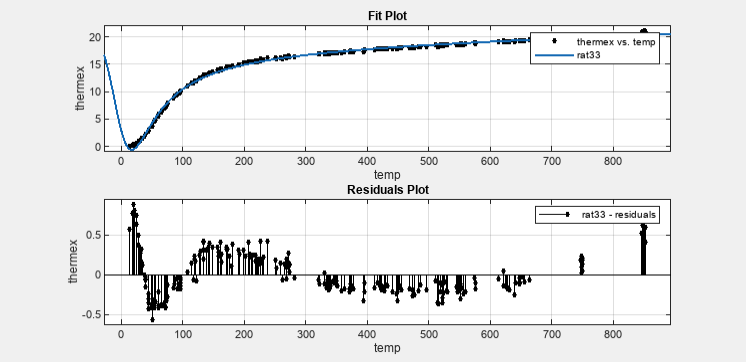8. Look in the Results pane. The message and numerical results indicate that the fit did not converge.

```Fit computation did not converge: Fitting stopped because the number of iterations or function evaluations exceeded the specified maximum. ```

Although the message in the Results pane indicates that you might improve the fit if you increase the maximum number of iterations, a better choice at this stage of the fitting process is to use a different rational equation because the current fit contains several discontinuities. These discontinuities are due to the function blowing up at predictor values that correspond to the zeros of the denominator.

9. Try fitting the data using a cubic/quadratic equation. Select `2` for the Denominator degree and leave the Numerator degree set to `3`.

10. The input variables have very different scales, so select the Center and scale option.

The data, fit, and residuals are shown below.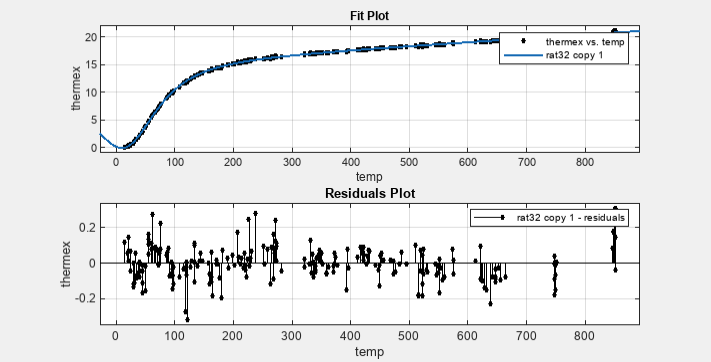The fit is well behaved over the entire data range, and the residuals are randomly scattered about zero. Therefore, you can confidently use this fit for further analysis.